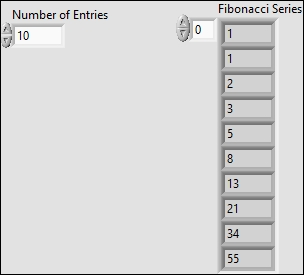# Generating Fibonacci Series with LabVIEW

Fibonacci series can be generated with LabVIEW using a For Loop, Shift Registers and an Addition Operator.
In the example below, we provide a Front Panel Control with the number of Fibonacci series entries to generate.
We initialize two shift registers, and pass the current Fibonacci sum as well as the previous Fibonacci entry to the next iteration. This allows us to calculate the next Fibonacci series value. The output tunneling mode of the Additional operator is set to Indexed so we can store the Fibonacci series numbers in an Array.Finally, we display the Fibonacci series on the Front Panel using an Array output Indicator